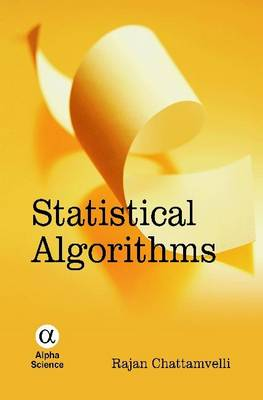•# Statistical Algorithms (Hardback)

(author)
£29.95
Hardback 304 Pages / Published: 09/03/2012
• We can order this

Usually dispatched within 1 week

STATISTICAL ALGORITHMS integrates up-to-date theoretical and algorithmic aspects of statistics under one roof. Starting with elementary algorithms on mean, median and mode, it thoroughly discusses variance, covariance, correlation, skewness and kurtosis measures, distance metrics, regression models, and variable selection methods. The chapter on matrix algorithms summarises a large number of useful results. Algorithms for the most popular discrete and continuous statistical distributions appear in chapters 9 and 10. Estimation in a missing data setup is numerically exemplified in the chapter on Expectation Maximisation (EM) algorithm. Random number generation and Monte Carlo methods are also discussed. A key feature of the book is the large number of code-snippets and pseudocode of algorithms. No prior knowledge in statistics or mathematics is assumed on the part of the reader, but only basic knowledge in computer program coding in any high-level language. This book is an invaluable resource for undergraduate students, statisticians and applied mathematicians, computer scientists, engineers and professionals working in related fields.

Publisher: Alpha Science International Ltd
ISBN: 9781842657270
Number of pages: 304
Dimensions: 240 x 160 x 26 mm